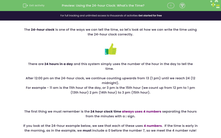# Use the 24-hour Clock to Calculate the Time

In this worksheet, students will add or subtract minutes to or from given times, stating their answers in 24-hour clock time.Key stage:  KS 2

Curriculum topic:   Measurement

Curriculum subtopic:   Solve Time Problems

Difficulty level:#### Worksheet Overview

The 24-hour clock is one of the ways we can tell the time, so let's look at how we can write the time using the 24-hour clock correctly.There are 24 hours in a day and this system simply uses the number of the hour in the day to tell the time.

After 12:00 pm on the 24-hour clock, we continue counting upwards from 13 (1 pm) until we reach 24 (12 midnight).

For example: 11 am is the 11th hour of the day, and 3 pm is the 15th hour (we count up from 12 pm to 1 pm (13th hour) 2 pm (14th hour) to 3 pm (15th hour).

Alternatively, you can add 12 to the hours to work out the time after 12 noon.

For example: 04:00 (+ 12) = 16:00 and 07:00 (+ 12) = 19:00

The first thing we need to remember is that the 24 hour clock time always uses four numbers, separating the hours from the minutes with a : sign.

If you look at the 24-hour example below, we see that each of these uses four numbers.  If the time is early in the morning, as in the example, we must include a 0 before the number 7, so we meet the four number rule!

7.35 a.m. is 07:35

7.35 p.m. is 19:35

You may notice that when we write the time using the 24-hour clock, we don't write am or pm.  This is because we know that anything between 00:00 and 11:59 (the first 12 hours) refers to the morning, and 12:00 - 23:59 is the afternoon/evening.

Once we know these basic rules for writing the time, using the 24-hour clock, we can start to do more advanced things, like adding and subtracting time. Let's give this a go!

Example

What 24-hour clock time is 46 minutes after 7.35 p.m.?

We start on the hour at 7.00 p.m. and then add 46 minutes and 35 minutes from the question.

35 + 46 = 81 minutes.

81 minutes is 1 hour (60 minutes) and 21 minutes.

1 hour and 21 minutes after 7.00 p.m. is 8.21 p.m.

8.21 p.m. is 20:21 in 24 hour clock time.